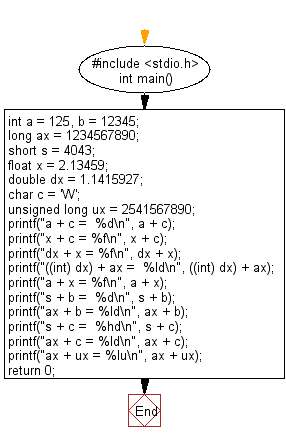﻿ C Program: Display multiple variables - w3resource

# C Exercises: Display multiple variables

## C Basic Declarations and Expressions: Exercise-7 with Solution

Write a C program to display following variables.
a+ c, x + c,dx + x, ((int) dx) + ax, a + x, s + b, ax + b, s + c, ax + c, ax + ux
Variable declaration :
int a = 125, b = 12345;
long ax = 1234567890;
short s = 4043;
float x = 2.13459;
double dx = 1.1415927;
char c = 'W';
unsigned long ux = 2541567890;

C Code:

``````#include <stdio.h>

int main()
{
int a = 125, b = 12345;        /* Declare and initialize integer variables */
long ax = 1234567890;          /* Declare and initialize long integer variable */
short s = 4043;                /* Declare and initialize short integer variable */
float x = 2.13459;             /* Declare and initialize floating-point variable */
double dx = 1.1415927;         /* Declare and initialize double precision variable */
char c = 'W';                  /* Declare and initialize character variable */
unsigned long ux = 2541567890; /* Declare and initialize unsigned long integer variable */

/* Various arithmetic operations and type conversions */
printf("a + c =  %d\n", a + c);
printf("x + c = %f\n", x + c);
printf("dx + x = %f\n", dx + x);
printf("((int) dx) + ax =  %ld\n", ((int) dx) + ax);
printf("a + x = %f\n", a + x);
printf("s + b =  %d\n", s + b);
printf("ax + b = %ld\n", ax + b);
printf("s + c =  %hd\n", s + c);
printf("ax + c = %ld\n", ax + c);
printf("ax + ux = %lu\n", ax + ux);

return 0;
}
``````

Sample Output:

```a + c =  212
x + c = 89.134590
dx + x = 3.276183
((int) dx) + ax =  1234567891
a + x = 127.134590
s + b =  16388
ax + b = 1234580235
s + c =  4130
ax + c = 1234567977
ax + ux = 3776135780
```

Flowchart:C Programming Code Editor:

What is the difficulty level of this exercise?

Test your Programming skills with w3resource's quiz.

﻿